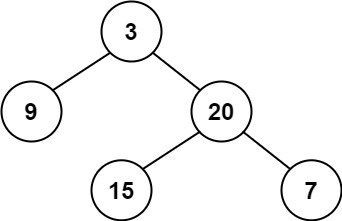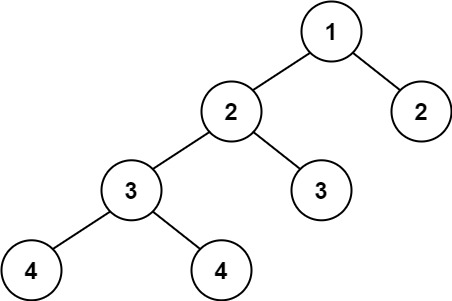# GeetCode Hub

Given a binary tree, determine if it is height-balanced.

For this problem, a height-balanced binary tree is defined as:

a binary tree in which the left and right subtrees of every node differ in height by no more than 1.

Example 1:```Input: root = [3,9,20,null,null,15,7]
Output: true
```

Example 2:```Input: root = [1,2,2,3,3,null,null,4,4]
Output: false
```

Example 3:

```Input: root = []
Output: true
```

Constraints:

• The number of nodes in the tree is in the range `[0, 5000]`.
• `-104 <= Node.val <= 104`

/** * Definition for a binary tree node. * public class TreeNode { * int val; * TreeNode left; * TreeNode right; * TreeNode() {} * TreeNode(int val) { this.val = val; } * TreeNode(int val, TreeNode left, TreeNode right) { * this.val = val; * this.left = left; * this.right = right; * } * } */ class Solution { public boolean isBalanced(TreeNode root) { } }